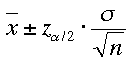The following Applet simulates taking a random sample of the 1997 Penny Data. A random selection of the 1534 pennies can approximate the actual mean year of 86.156 (i.e., a mean age of 10.844 years). The 95% confidence interval is represented by the blue line, with the sample mean at the red dot.

## Excercises

1. Take 100 samples of 5 pennies, and see how often the blue line does not touch the orange line (i.e., How often does is the actual mean excluded from the confidence interval?
2. Make the same ovservation of 100 samples of 200 pennies each.
3. What is different?
4. What is the same?
5. Experiment with different sample sizes. What seems to be the best estimate? It takes considerably longer to sample 500 pennies than 100; is the estimate worth the trouble?
6. What seems to effect the size of the confidence interval more: the standard deviation of the population of pennies, or the number of pennies in your random sample? (Look below the applet to see the formula for determiining the confidence interval of a mean)Fr. Chris' Java applet.